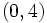# Flow of a metric

## Definition

### Definition with symbols

Let$M$ be a Riemannian manifold. A flow of a metric on$M$ is defined as a map from an interval (possibly infinite) in the real numbers, to the space of all possible Riemannian metrics on the manifold. The domain of this map is often called the time domain and we think of the Riemannian metric as evolving with time. We typically require the flow to be smooth, that is, the metric should vary smoothly as a function of time.

## Notions

### Invariants of a flow

We may require that the flow does not change a certain property, or quantity, associated with the metric. For instance, a conformal flow is a flow that does not change the conformal class of the metric (viz the metrics at any two points in time are conformally equivalent). A volume-normalized flow does not change the total volume of the manifold (viz at any two points in time, the total volume of the manifolds is the same).

### Goal of the flow

The goal of the flow may be to eventually reach a nice metric, such as:

and so on

A flow is said to terminate in finite time if it reaches its goal in finite time, that is, after a finite time, the metric stops evolving. A flow is said to converge if, in the limit, it reaches a particular kind of flow.

## Other things which evolve with the metric

### Riemann curvature tensor

The Riemann curvature tensor is a (1,3)-tensor (or a (0,4)-tensor) on the manifold, determined by the Levi-Civita connection, which is turn is determined by the Riemannian metric. Thus, as the Riemannian metric evolves, so does the curvature tensor. The curvature tensor flows along, or traces a path in, the space of$(0,4)$-tensors. In fact, since the Riemann curvature tensor always lives inside the Riemann curvature bundle (which is independent of the metric), we actually get a flow in the Riemann curvature bundle.

### Ricci curvature tensor

The Ricci curvature tensor is a (0,2)-tensor on the manifold, determined by the Riemann curvature tensor, which in turn is determined by the Levi-Civita connection, which in turn is determined by the Riemannian metric. Thus, as the metric evolves, so does the Ricci curvature tensor. Further, the Ricci curvature tensor is a tensor of the same type as the metric tensor, hence their evolution can be studied together (this is precisely what is done for the Ricci flow and the volume-normalized Ricci flow).

### Scalar curvature

As the Riemannian metric evolves, so does the scalar curvature, which is after all defined in terms of the Riemannian metric. The scalar curvature is an ordinary scalar function defined on the manifold. The evolution of scalar curvature is governed by a single equation as opposed to the multiple equations that govern the evolution of the Ricci flow and the volume-normalized Ricci flow.